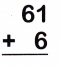Practice questions available in McGraw Hill Math Grade 2 Answer Key PDF Chapter 2 Lesson 1 Adding Through 99 will engage students and is a great way of informal assessment.

Write the sum.

Question 1.By adding 25 and 33 we get 58.

Question 2.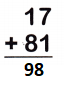By adding 17 and 81 we get 98.

Question 3.By adding 54 and 12 we get 66.

Question 4.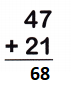By adding 47 and 21 we get 68.

Question 5.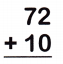By adding 72 and 10 we get 82.

Question 6.By adding 36 and 13 we get 49.

Question 7.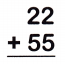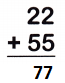By adding 22 and 55 we get 77.

Question 8.